Understanding how different loans work and how they affect your bottom line both now and in the future is the key to making solid financial decisions. There are a number of different types of loans available on the market today and choosing the right one for you can be complicated or even intimidating. Luckily, thanks to Excel, you can easily compare the effects that different loans will have on your finances both now and in the future.

You can use Excel to set up an amortization schedule with a balloon payment. You can create a spreadsheet to compare the different effects of different loans so that you can take control of your finances by making the right financial decisions. Jean Chatzky’s Money School: Jumpstart Your Finances course will help you get your finances back on track so that you can begin to build a secure financial future. The course will teach you about smart spending, how to supercharge your savings, how to conquer debt and how to manage your credit. The course offers a checklist that will show you exactly what to do next to achieve a solid financial future.

## Balloon Payment Loans

A balloon payment loan is a loan that does not fully amortize over the term of the loan. The payments therefore do not cover the loan entirely and at the end of the loan, a lump sum payment is required to settle the loan. Balloon loans are often seen as an attractive alternative to ordinary loans because the repayments are smaller in the short term, but buyers should be aware that the lump sum payment at the end of the loan carries refinancing risks that include refinancing the lump sum at a higher interest rate.

This blog will show you how to set up an amortization schedule with a balloon payment so that you can calculate the repayments and compare what the loan will actually cost you compared with other loans. You will need access to certain data to set up the worksheet. You will need to know the amount of the loan, the interest rate of the loan, the number of payments, the amount of the payments and amount of the balloon payment. Ultimately, the schedule will show you how much this loan will actually cost you. Knowing the cost of a loan is essential when you want to budget properly. The Budget – Save – Win. Simplify your financial life course offers a unique budgeting solution that will help you to simplify and improve your financial life.

## Create a New Worksheet

To create the worksheet, start by creating a new worksheet in Excel based on the following example: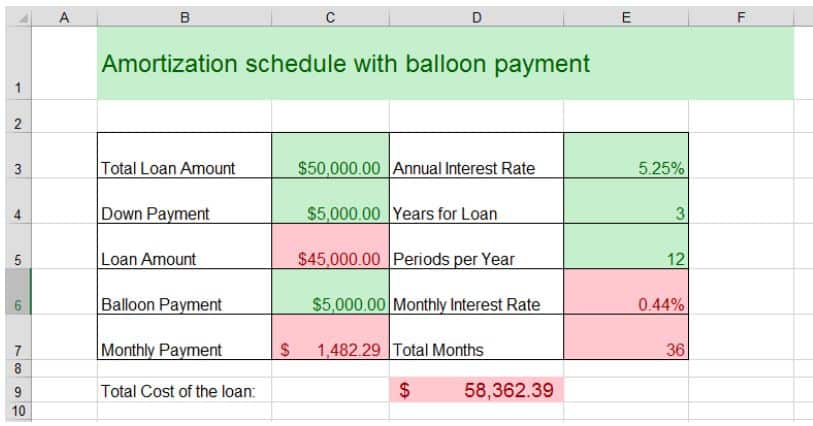The fields marked as green in the example will be filled in by the user when you are ready to compare loans. The red fields are based on formulas that you will add to your worksheet.

The Loan Amount is calculated by subtracting the Down Payment field from the Total Loan Amount field. The formula looks like this:

=C3-C4

The Monthly Payment Formula uses the PMT function in Excel and is used to calculate the payment due for the loan. The formula looks like this:

=-PMT(E6,E7,C5,C6)

The Payment function in Excel is great for calculating payments due on a loan. To access the payment function, you can either type in the formula above, or you can select the Monthly Payment Cell and then select “PMT” from the “Financial” formulas found in the Formulas menu.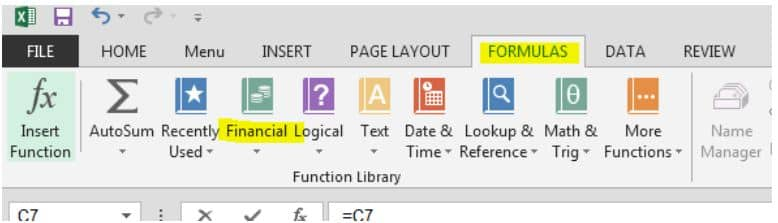When you select the PMT function, the function arguments window open automatically to allow you to enter the arguments for the function: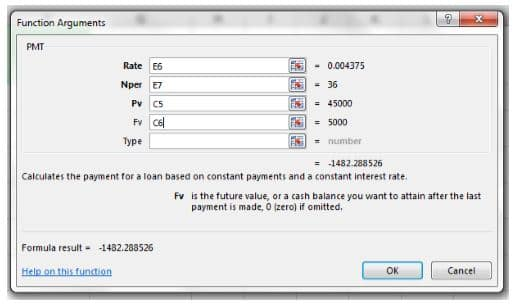The rate is the interest rate for the loan per payment period. In this case it is the monthly interest rate that applies to your loan. The NPer refers to the total number of payments for the loan. The PV represents the total loan amount and the FV in this case represents the balloon payment due at the end of the loan.

The monthly interest rate is calculated by dividing the annual interest rate by twelve. The formula looks like this:

=E3/E5

The total month is calculated by multiplying the years of the loan by the number of payment periods. The formula looks like this:

=E4*E5

Finally, the total cost of the loan is calculated by multiplying the monthly payment by the total months and then subtracting the balloon payment due:

=-(+C7*-E7-C6)

You now have a spreadsheet that will calculate your payments as well as the total amount you will pay for your loan. All you need to do is fill in the total loan amount, down payment amount, balloon payment amount, the interest rate, number of years and number of payments per year.

You can now add an amortization schedule to your worksheet to see the effects of monthly payments on the capital amount. To add an amortization schedule you need to add the column heading below: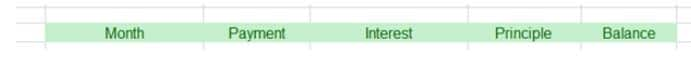You can add as many lines as you want to your schedule to represent the monthly payments. We Have 12 lines to represent the first year: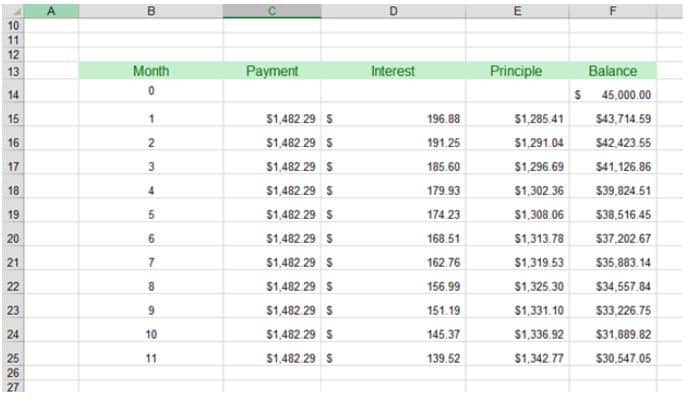In the first month, you need to create the starting balance of the loan by using the following formula:

=+C5

The payment amount column needs to set the payment amount equal to the payment calculated above so you need to use the following absolute formula for the payment amount:

=+\$C\$7

The interest should be calculated by multiplying the balance of the loan times the monthly interest rate:

=+F14*\$E\$6

The principle amount paid for that month is calculated by subtracting the interest portion of the payment made for that month:

=C15-D15

Finally the balance is calculated by subtracting the principle paid for that month from the principle amount outstanding.

Now you can add as many lines as you want to view your amortization schedule.

## Compare Your Loans and Learn To Budget

The key to a successful and prosperous financial future lies in learning to budget and comparing loans to find the best value loan for you. Jean Chatzky’s Money School: Budgeting Bootcamp will teach you how to live below your means so that you can save, pay down your debts and so that you can invest in your future financial success.

Page Last Updated: April 2014

### Top courses in Excel

Microsoft Excel - Excel from Beginner to Advanced
Kyle Pew, Office Newb
4.6 (400,246)
Bestseller
Microsoft Excel: Data Analysis with Excel Pivot Tables
Maven Analytics, Chris Dutton
4.6 (55,191)
Bestseller
Microsoft Excel: Data Visualization, Excel Charts & Graphs
Maven Analytics, Chris Dutton
4.6 (42,917)
Bestseller
Microsoft Excel Pro Tips: Go from Beginner to Advanced Excel
Maven Analytics, Chris Dutton
4.6 (4,965)
Bestseller
Microsoft Excel: Beginner to Advanced for 2023
4.6 (2,207)
Microsoft Excel - Excel from Beginner to Advanced 2023
Warrick Klimaytys
4.6 (8,041)
Bestseller
Microsoft Excel Certification Exam Prep: MO-201 Excel Expert
Maven Analytics, Enrique Ruiz
4.7 (1,888)
Bestseller